# 5 Top-Of-The-Line Plays: 3 Excellent And 2 Good

|
Includes: CVS, FRT, GWW, KMP, UPS
by: Tactical Investor

"Never feel self-pity, the most destructive emotion there is. How awful to be caught up in the terrible squirrel cage of self." -- Millicent Fenwick

Novice investors tend to base their decisions on yield alone: while it's okay to risk a small amount of one's capital using this strategy, betting the house is asking for trouble. Our picks (especially our favorite play) have to generally meet most of the criteria listed below.

Net income: it should be generally trending upwards for the past 3-4 years.

Total cash flow from operating activities: it also should be trending upwards for the past 3-4 years.

Payout ratio: it should generally be below 100%, but a ratio below 70% is optimal. Payout ratios are not that important when it comes to MLPS/REITS as they generally pay a majority of their cash flow as distributions; in the case of REITS by law they have to pay out 90% of their cash flow as dividends. Payout ratios are calculated by dividing the dividend/distribution rate by the net income per share, and this is why the payout ratio for MLPs and REITS is often higher than 100%. The more important ratio to focus on is the cash flow per unit. If one focuses on the cash flow per unit, one will see that in most cases, it exceeds the distribution/dividend declared per unit/share.

Current ratio: should be above 1

Interest coverage ratio: any value above 1.5 is okay, but we would aim for 2.5-3.00 as our starting range. The higher the number the better

Dividend growth rate: it should be at 5% or higher. A high yield with a low dividend growth rate is not good in the long run, but neither is a low dividend yield with a high growth rate; one needs to find an equilibrium here.

Five year dividend average: we generally aim for stocks that have a yield of 4.5% or higher. There are exceptions to this rule. Some stocks appreciate very fast, so even though the yield might be low, one can more than make up the difference through capital gains. One example is JAH.

Sales: they should generally be trending upwards for the past 3-4 years.

Levered free cash flow: this is the icing on the cake; if a company meets most of the above requirements and also has a positive levered free cash flow, it can generally be viewed as a good long term buy. Two examples are LEG and PG.

An early warning signal that the company could be in trouble is when the total cash flow generated from operating expenses is not enough to meet the dividend payments. This information can be gleaned by looking at the cash flow statement; this is readily available at yahoo finance. In the example below we used LEG and the data was obtained from Yahoo Finance.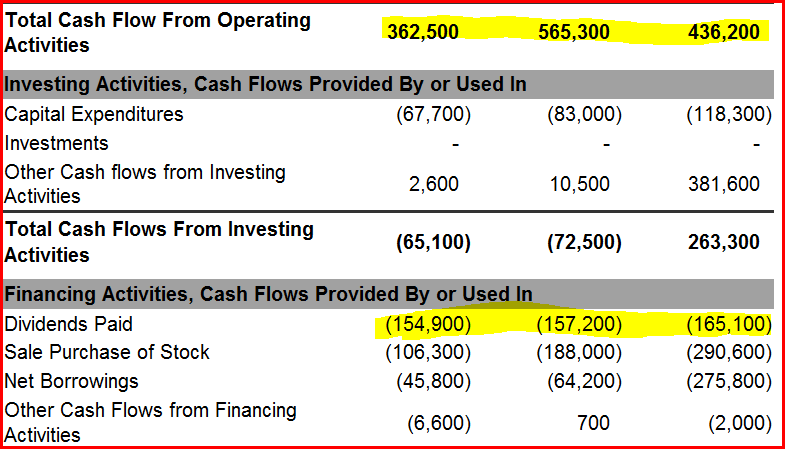The cash flow in this case was more than enough to easily cover all the dividend payments for all the above years; in this the time period was from 2008-2010.

Many traders use other metrics, and that is fine; we are just trying to provide a guideline. As you get better handle of the ratios explained below, you can create your own list of criteria.

Long-term debt-to-equity ratio is the total long term debt divided by the total equity. The amount of long-term debt a company carries on its balances sheet is very important for it indicates the amount of money a company owes that it doesn't expect to pay off in the next year. A balance sheet that illustrates that long term debt has been decreasing for a few years is a sign that the company is doing well. When debt levels fall, and cash levels increase the balance sheet is said to be improving and vice versa. If a company has too much debt on its books, it could end up being overwhelmed with interest payments and risk having too little working capital which could in the worst case scenario lead to bankruptcy.

Operating cash flow is generally a better metric than earnings per share because a company can show positive net earnings and still not be able to properly service its debt; the cash flow is what pays the bills.

The payout ratio tells us what portion of the profit is being returned to investors. A pay out ratio over 100% indicates that the company is paying out more money to shareholders than they are making; this situation cannot last forever. In general if the company has a high operating cash flow and access to capital markets, they can keep this going on for a while. As companies usually only pay the portion of the debt that is coming due and not the whole debt, this technique/trick can technically be employed to maintain the dividend for sometime. If the payout ratio continues to increase, the situation warrants close monitoring. If your tolerance for risk is a low, look for similar companies with the same or higher yields, but with lower payout ratios. Individuals searching for other ideas might find this article to be of interest 5 Growth Plays To Consider: 2 Great, 1 Good And 2 Mediocre.

Current Ratio is obtained by dividing the current assets by current liabilities. This ratio allows you to see if the company can pay its current debts without potentially jeopardizing their future earnings. Ideally the company should have a ratio of 1 or higher.

Price to free cash flow is obtained by dividing the share price by free cash flow per share. Higher ratios are associated with more expensive companies and vice versa; lower ratios are generally more attractive. If a company generated 400 million in cash flow and then spent 100 million on capital expenditure, then its free flow is \$300 million. If the share price is 100 and the free cash flow per share are \$5, then company trades at 20 times-free cash flow. This ratio is also useful because it can be used as a comparison to the average within the industry; this gives you an idea of how the company you are interested in holds up to the other companies within the industry.

Interest coverage is usually calculated by dividing the earnings before interest and taxes for a period of 1 year by the interest expenses for the same time period. This ratio informs you of a company's ability to make its interest payments on its outstanding debt. Lower interest coverage ratios indicate that there is a larger debt burden on the company and vice versa. For example, if a company has an interest ratio of 11.8, this means that it covers interest expenses 11.8 times with operating profits.

Price to tangible book is obtained by dividing share price by tangible book value per share. The ratio gives investors some idea of whether they are paying too much for what would be left over if the company were to declare bankruptcy immediately. In general stocks that trade at higher price to tangible book value could leave investors facing a great percentage per share loss than those that trade at lower ratios. The price to tangible book value is theoretically the lowest possible price the stock would trade to. Additional key metrics are addressed in this article 5 Interesting Candidates With Good Growth Potential

Federal Realty Investment Trust (NYSE:FRT) is our play of choice for the following reasons:

• A good free cash flow of \$199 million
• Net income increased from \$98 million in 2009 to \$144 million in 2011.
• Gross profit increased from \$362 million in 2009 to \$382 million in 2011.
• Total cash flow from operating activities is more than enough to cover dividend payments.
• Has a manageable payout ratio of 69%?
• It is a true dividend king for it has raised its dividend consecutively for 44 years.
• It has a very strong total three year return of 142%
• Even though it has a relatively low yield it has more than made up for this via capital gains. 100K invested in FRT for 10 years has grown to 496K.
• The low current and quick ratios of are of some concern; however, it does sport an average interest coverage ratio 2.24. If the interest coverage ratio drops to or below 1 we would start to become worried.
• Total Debt to equity is below 1; it currently stands at 0.87.
• Revenue is projected to increase from \$545 million in 2010 to \$602 million in 2013.
• Funds from operations per share are projected to increase from \$3.88 in 2010 to \$4.46 in 2013.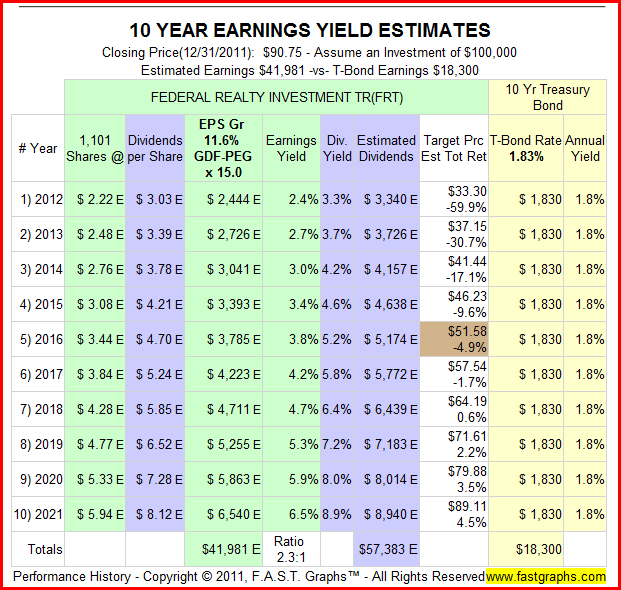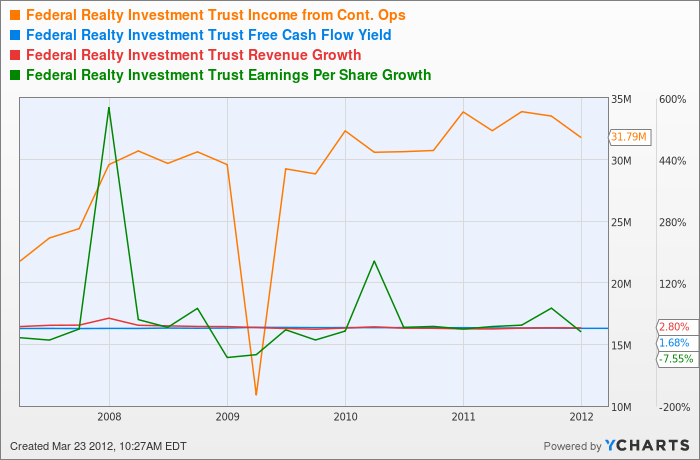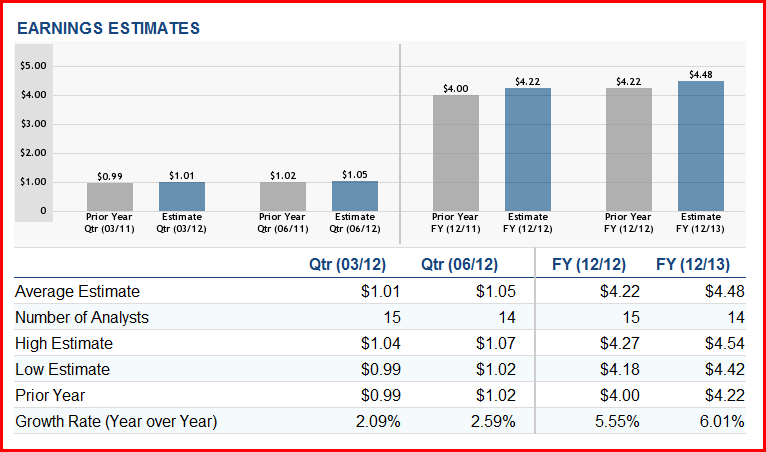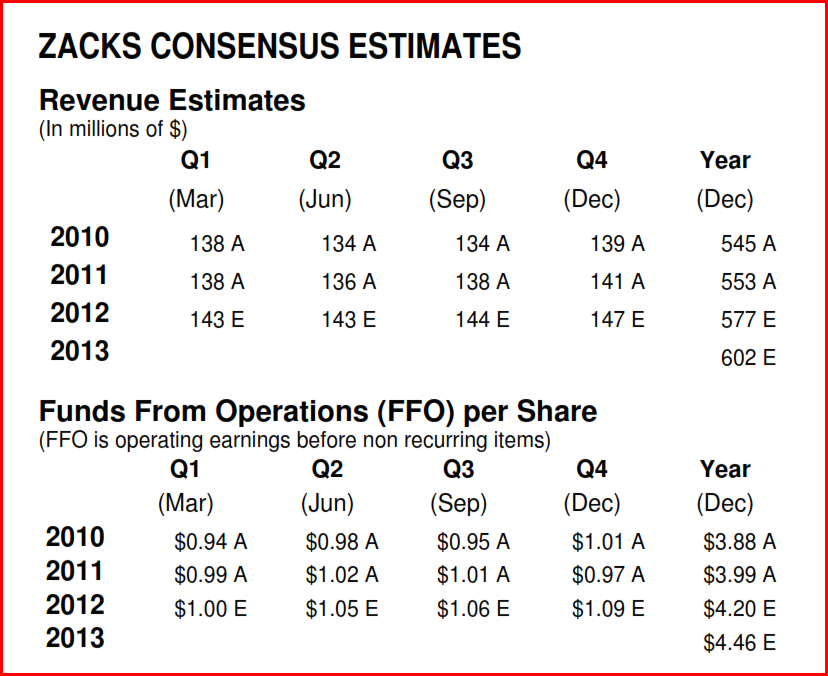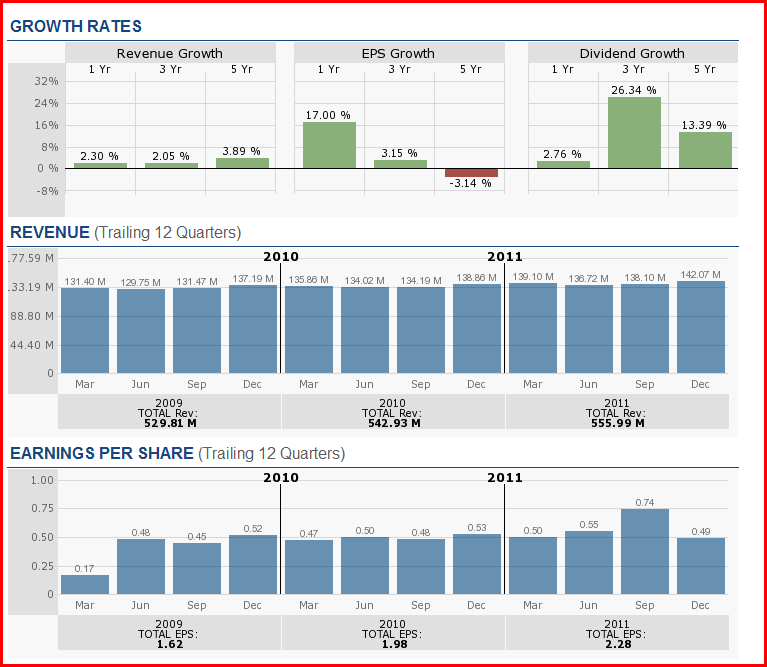### Federal Realty Investment Trust

Industry: REITs

Free Cash Flow: \$199 million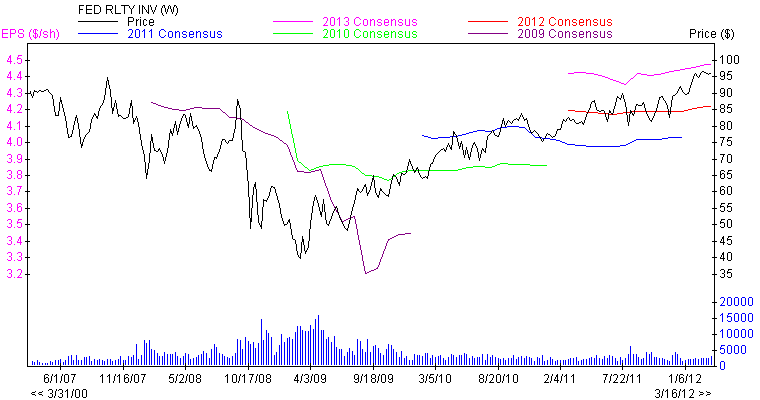Growth

1. Net income for the past three years
2. Net Income (\$mil) 2009 = \$98
3. Net Income (\$mil) 2010 = \$123
4. Net Income (\$mil) 2011 = \$144
1. Total cash flow from operating activities
2. 2009 = \$256.77 million
3. 2010 = \$256.74 million
4. 2011 = \$244.72 million
1. Cash And Cash Equivalents for the past 3 years
2. 2009= \$135.3 million
3. 2010= \$15.7 million
4. 2011= \$67.8 million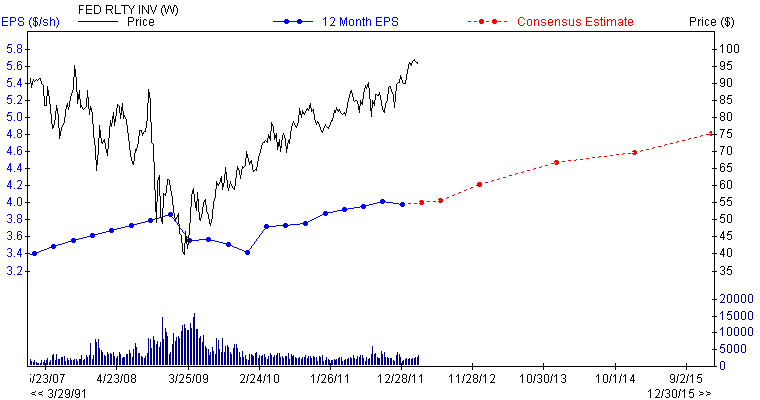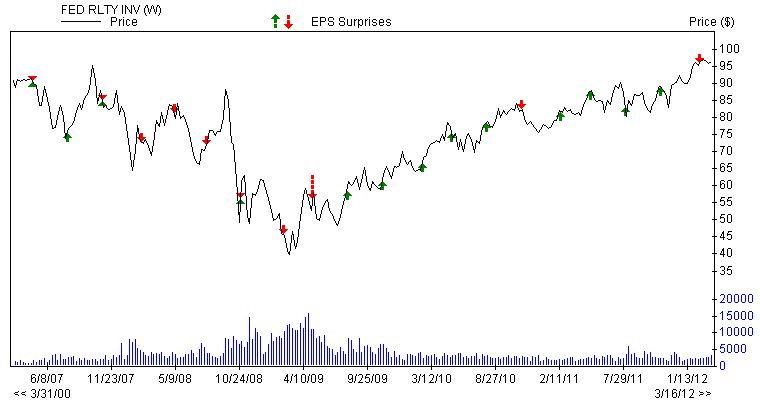Performance

1. Key Ratios
2. Price to Sales = 11.13
3. Price to Book = 5.09
4. Price to Tangible Book = 5.09
5. Price to Cash Flow = 41.20
6. Price to Free Cash Flow = 403
1. LT Debt to Equity = 1.75
2. Total Debt to Equity = 0.87
3. Interest Coverage = 2.24
4. Asset Turnover = 0.15
1. ROE = 10.50%
2. Return on Assets = 4.2%
3. Quarterly Earnings Growth = -5.8%

Dividend history and sustainability

1. Dividend yield 5 year average = 3.54%
2. Payout ratio = 0.69
3. Dividend growth rate 3 year average = 2.8%
4. Dividend growth rate 5 year average = 3.7%
5. Consecutive dividend increases = 44 years
6. Total return last 3 years = 149%
7. Total return last 5 years = 13%

Notes

FRT falls under the category of "excellent"

### Kinder Morgan Energy (NYSE:KMP)

Free Cash Flow = \$1.67 billion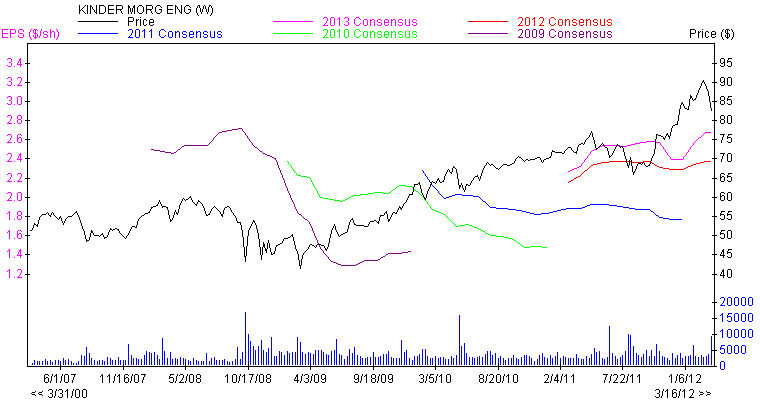Basic Key ratios

1. Percentage Held by Insiders = 20.72
2. Market Cap (\$mil) = 20804

Growth

1. Net Income (\$mil) 12/2011 = 1268
2. Net Income (\$mil) 12/2010 = 1316
3. Net Income (\$mil) 12/2009 = 1268
1. EBITDA (\$mil) 12/2011 = N/A
2. EBITDA (\$mil) 12/2010 = 2780
3. EBITDA (\$mil) 12/2009 = 2628
1. Cash Flow (\$/share) 12/2011 = 11.72
2. Cash Flow (\$/share) 12/2010 = 10.34
3. Cash Flow (\$/share) 12/2009 = 10.54
1. Sales (\$mil) 12/2011 = 8211
2. Sales (\$mil) 12/2010 = 8078
3. Sales (\$mil) 12/2009 = 7003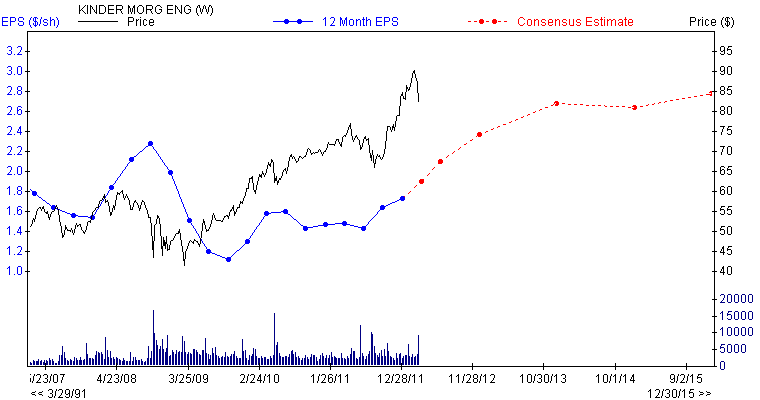Dividend history

1. Div Yield = 5.60
2. Div Yield 5 Yr Average 12/2011 = 6.8
3. Annual Dividend 12/2011 = 4.58
4. Forward Yield = 5.15
5. Div 5yr Growth 12/2011 = 7.31

Dividend sustainability

1. Payout Ratio 09/2011 = 2.81
2. Payout Ratio 5 Yr Average 12/2011 = 2.62
3. Change in Payout Ratio = 0.07

Performance

1. 5 Yr Historical EPS Growth 12/2011 = -3.7
2. ROE 5 Yr Average 12/2011 = 22.23
3. Return on Investment 12/2011 = 9.37
4. Debt/Tot Cap 5 Yr Average 12/2011 = 60.61
1. Current Ratio 12/2011 = 0.44
2. Current Ratio 5 Yr Average = 0.54
3. Quick Ratio = 0.41
4. Cash Ratio = 0.15
5. Interest Coverage 12/2011 = 5.26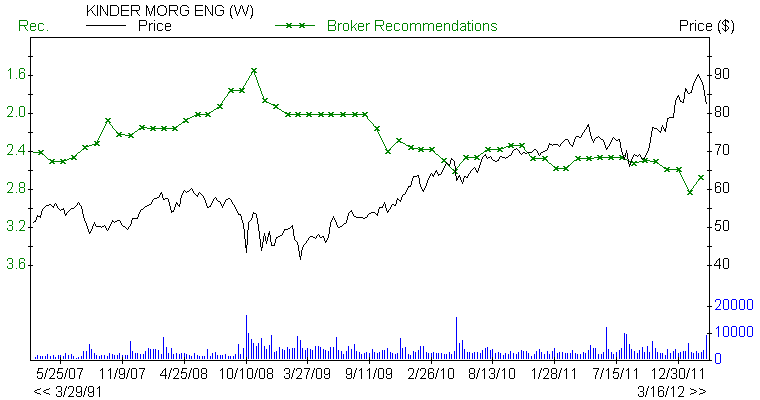Valuation

1. Book Value Qtr (\$/sh) 12/2011 = 32.93
2. Book Value Qtr (\$/sh) 09/2011 = 33.69
3. Book Value Qtr (\$/sh) 06/2011 = 33.76
4. Anl EPS before NRI 12/2007 = 1.74
5. Anl EPS before NRI 12/2008 = 2.07
6. Anl EPS before NRI 12/2009 = 1.31
7. Anl EPS before NRI 12/2010 = 1.47
8. Anl EPS before NRI 12/2011 = 1.75
1. Price/ Book = 2.74
2. Price/ Cash Flow = 7.69
3. Price/ Sales = 2.53
4. EV/EBITDA 12 Mo = 11.17
5. P/E/G F1 = 7.57

Notes

It falls under the category of "excellent."

### United Parcel Service Inc (NYSE:UPS)

Industry: Airlines/Air Freight

Levered Free Cash Flow : 4.28B

Growth

1. Net income for the past three years
2. Net Income 2009 = \$1968 million
3. Net Income 2010 = \$3338 million
4. Net Income 2011 = \$3804 million
1. EBITDA 12/2011 = \$7906 million
2. EBITDA 12/2010 = \$7436 million
3. EBITDA 12/2009 = \$5265 million
4. Net income Reported Quarterly = \$1690 million
1. Total cash flow from operating activities
2. 2009 = \$5.29 billion
3. 2010 = \$3.84 billion
4. 2011 = \$7.08 billion
1. Cash Flow 12/2011 = 6.31 \$/share
2. Cash Flow 12/2010 = 5.42 \$/share
3. Cash Flow 12/2009 = 4.09 \$/share
1. Annual EPS before NRI 12/2011 = 4.35
2. Annual EPS before NRI 12/2010 = 3.56
3. Annual EPS before NRI 12/2009 = 2.31
4. Annual EPS before NRI 12/2008 = 3.5
5. Annual EPS before NRI 12/2007 = 4.17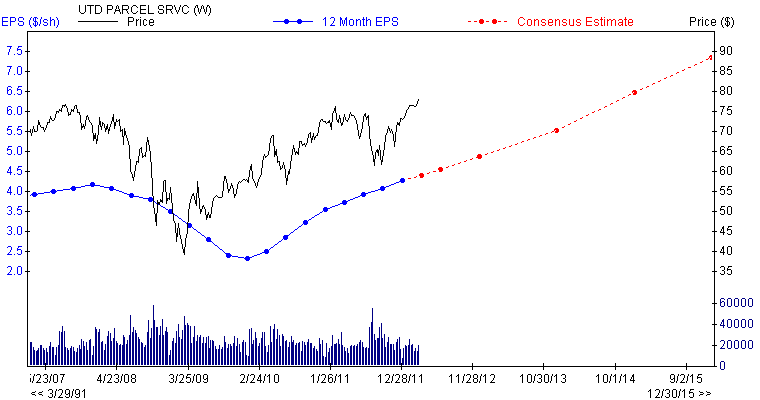Performance

1. ROE = 53.55%
2. Return on Assets = 12.09%
3. Quarterly Earnings Growth = -16%
4. Quarterly Revenue Growth = 5.6%
5. Price to Sales = 1.43
6. Price to Book = 10.72
7. Price to Tangible Book = 17.4
8. Price to Cash Flow = 12.5
9. Price to Free Cash Flow = 25.2
1. Current Ratio 09/2011 = 1.89
2. Current Ratio 5 Year Average = 1.41
3. Quick Ratio = 1.89
4. Cash Ratio = 0.93
5. Interest Coverage 09/2011 = 20.68

Dividend history and sustainability

1. Payout Ratio 09/2011 = 0.49
2. Payout Ratio 06/2011 = 0.51
3. Payout Ratio 5 Year Average 09/2011 = 0.55
4. Payout Ratio 5 Year Average 06/2011 = 0.54
5. Change in Payout Ratio = -0.06
1. Dividend yield 5 year average = 3%
2. Dividend growth rate 3 year average = 5.84%
3. Dividend growth rate 5 year average = 6.48%
4. Consecutive dividend increases = 2 years
5. Paying dividends since = 1999
6. Total return last 3 years = 92%
7. Total return last 5 years = 26%

Notes

It falls under the category of "good-excellent"

### Grainger W W (NYSE:GWW)

Levered Free Cash Flow = 479.64M

Basic Key ratios

1. Percentage Held by Insiders = 8.05
2. Market Cap (\$mil) = 15176
3. Number of Institutional Sellers 12 Weeks = 2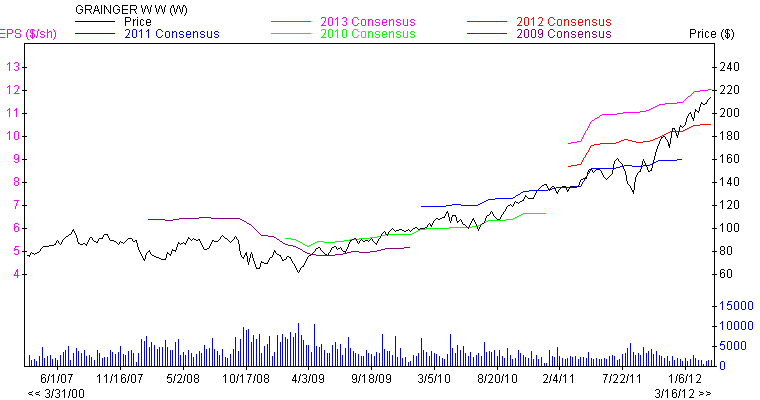Growth

1. Net Income (\$mil) 12/2011 = 658
2. Net Income (\$mil) 12/2010 = 511
3. Net Income (\$mil) 12/2009 = 430
4. 12months Net Income this Quarterly/ 12months Net Income 4Q's ago = 30.13
5. Quarterly Net Income this Quarterly/ same Quarter year ago = 12.31
1. EBITDA (\$mil) 12/2011 = 1210
2. EBITDA (\$mil) 12/2010 = 1012
3. EBITDA (\$mil) 12/2009 = 864
4. Net Income Reported Quarterlytr (\$mil) = 148
5. Annual Net Income this Yr/ Net Income last Yr = 28.89
1. Cash Flow (\$/share) 12/2011 = 11.55
2. Cash Flow (\$/share) 12/2010 = 9.44
3. Cash Flow (\$/share) 12/2009 = 7.4
1. Sales (\$mil) 12/2011 = 8078
2. Sales (\$mil) 12/2010 = 7182
3. Sales (\$mil) 12/2009 = 6222
1. Annual EPS before NRI 12/2007 = 4.94
2. Annual EPS before NRI 12/2008 = 6.16
3. Annual EPS before NRI 12/2009 = 5.38
4. Annual EPS before NRI 12/2010 = 6.81
5. Annual EPS before NRI 12/2011 = 9.04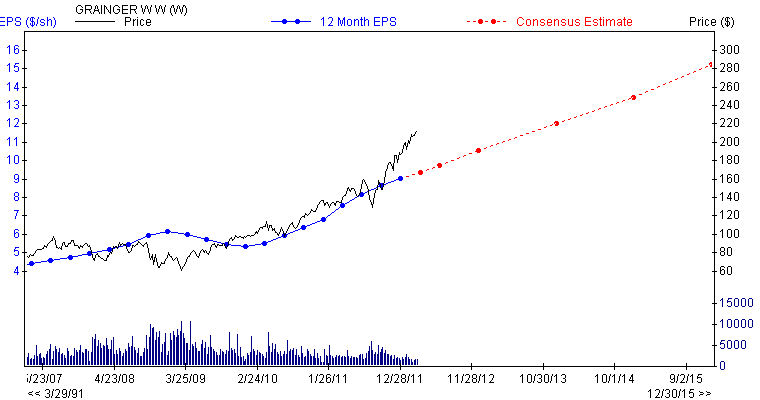Dividend history

1. Dividend Yield = 1.2
2. Dividend Yield 5 Year Average = 1.7%
3. Annual Dividend 12/2011 = 2.52
4. Annual Dividend 12/2010 = 2.08
5. Forward Yield = 1.22
6. Dividend 5 year Growth = 17%

Dividend sustainability

1. Payout Ratio 06/2011 = 0.29
2. Payout Ratio 5 Year Average 06/2011 = 0.3
3. Change in Payout Ratio = -0.01

Performance

1. Percentage Change Price 52 Weeks Relative to S&P 500 = 46.11
2. Next 3-5 Year Estimate EPS Growth rate = 13.4
3. EPS Growth Quarterly(1)/Q(-3) = -118.99
4. ROE 5 Year Average 06/2011 = 21.34
5. Return on Investment 06/2011 = 23.27
6. Debt/Total Cap 5 Year Average 06/2011 = 11.31
1. Current Ratio 06/2011 = 1.94
2. Current Ratio 5 Year Average = 2.66
3. Quick Ratio = 1.03
4. Cash Ratio = 0.39
5. Interest Coverage Quarterly = 85.68

Valuation

1. Book Value Quarterly = 39.06
2. Price/ Book = 5.54
3. Price/ Cash Flow = 18.74
4. Price/ Sales = 1.88
5. EV/EBITDA 12 Mo = 12.41

Notes

Grainger would fall under the category of "excellent."

### CVS Caremark (NYSE:CVS)

Levered Free Cash Flow = 4.00B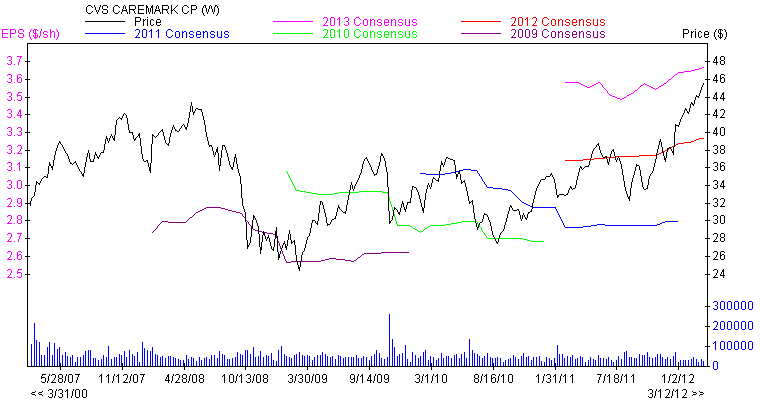Basic Key ratios

1. Percentage Held by Insiders = 0.94
2. Market Cap (\$mil) = 59441
3. Number of Institutional Sellers 12 Weeks = 1

Growth

1. Net Income (\$mil) 12/2011 = 3461
2. Net Income (\$mil) 12/2010 = 3427
3. Net Income (\$mil) 12/2009 = 3696
1. 12months Net Income this Quarterly/ 12months Net Income 4Q's ago = 0.99
2. Quarterly Net Income this Quarterly/ same Quarter year ago = 3.7
1. EBITDA (\$mil) 12/2011 = 7898
2. EBITDA (\$mil) 12/2010 = 7606
3. EBITDA (\$mil) 12/2009 = 7814
4. Net Income Reported Quarterlytr (\$mil) = 1064
5. Annual Net Income this Yr/ Net Income last Yr = 0.99
6. Cash Flow (\$/sh) 12/2011 = 4.1
7. Cash Flow (\$/sh) 12/2010 = 3.81
8. Cash Flow (\$/sh) 12/2009 = 3.8
1. Sales (\$mil) 12/2011 = 107100
2. Sales (\$mil) 12/2010 = 96413
3. Sales (\$mil) 12/2009 = 98729
1. Annual EPS before NRI 12/2007 = 2.07
2. Annual EPS before NRI 12/2008 = 2.44
3. Annual EPS before NRI 12/2009 = 2.74
4. Annual EPS before NRI 12/2010 = 2.69
5. Annual EPS before NRI 12/2011 = 2.8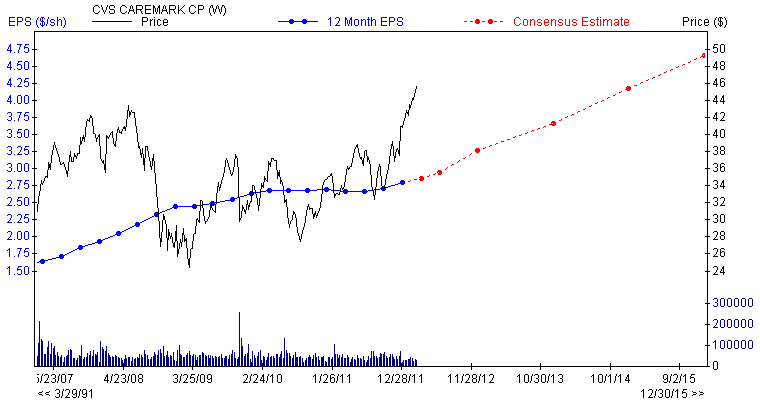Dividend history

1. Dividend Yield = 1.42
2. Dividend Yield 5 Year Average = 1.00%
3. Annual Dividend 12/2011 = 0.5
4. Annual Dividend 12/2010 = 0.35
5. Forward Yield = 1.42
6. Dividend 5 year Growth = 23%

Dividend sustainability

1. Payout Ratio 06/2011 = 0.18
2. Payout Ratio 5 Year Average 06/2011 = 0.14
3. Change in Payout Ratio = 0.04

Performance

1. Percentage Change Price 52 Weeks Relative to S&P 500 = 27.72
2. Next 3-5 Year Estimate EPS Growth rate = 11.83
3. EPS Growth Quarterly(1)/Q(-3) = -111.25
4. ROE 5 Year Average 06/2011 = 9.97
5. Debt/Total Cap 5 Year Average 06/2011 = 19.22
1. Current Ratio 06/2011 = 1.56
2. Current Ratio 5 Year Average = 1.44
3. Quick Ratio = 0.72
4. Cash Ratio = 0.21
5. Interest Coverage Quarterly = 13.23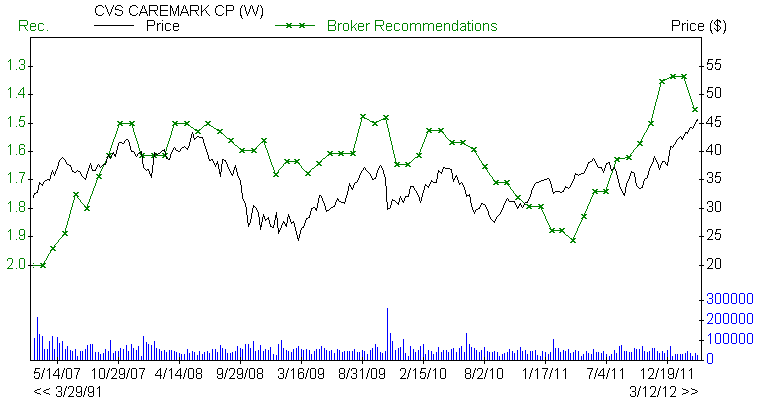Valuation

1. Book Value Quarterly = 29.23
2. Price/ Book = 1.56
3. Price/ Cash Flow = 11.14
4. Price/ Sales = 0.55
5. EV/EBITDA 12 Mo = 8.51

Notes

It falls under the category of "good."

Conclusion

The markets are extremely overbought right now. What makes the situation worse is the fact that the market is rising up on low volume and as of late the volume on down days has been inching up. Long-term investors would do well to wait for a nice decent pullback before deploying new sums of money into this market. If you are bullish on certain stocks, then you can sell naked put options near strike prices where you would not mind owning the stock. For example, if you like stock at 55 and it is now trading at 67, then you can sell naked puts with a strike of 55.

EPS, EPS surprise, broker recommendations, and price and consensus charts sourced from zacks.com. Earning's estimates and growth rate charts sourced from dailyfinance.com. Free cash flow yield, income from cont operations, and revenue growth sourced from Ycharts.com.

Disclosure: I have no positions in any stocks mentioned, and no plans to initiate any positions within the next 72 hours.

Disclaimer: This list of stocks is meant to serve as a starting point. Please do not treat this as a buying list. It is imperative that you do your due diligence and then determine if any of the above plays meet with your risk tolerance levels. The Latin maxim caveat emptor applies -- let the buyer beware.